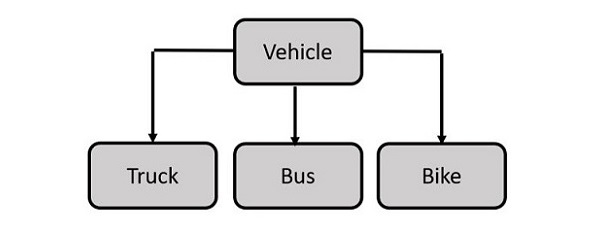# Python - Inheritance

Inheritance is one of the most important features of Object-oriented programming methodology. It is most often used in software development process using many languages such as Java, PHP, Python, etc.

Instead of starting from scratch, you can create a class by deriving it from a pre-existing class by listing the parent class in parentheses after the new class name.

Instead of starting from scratch, you can create a class by deriving it from a pre-existing class by listing the parent class in parentheses after the new class name.

If you have to design a new class whose most of the attributes are already well defined in an existing class, then why redefine them? Inheritance allows capabilities of existing class to be reused and if required extended to design new class.

Inheritance comes into picture when a new class possesses 'IS A' relationship with an existing class. Car IS a vehicle. Bus IS a vehicle; Bike IS also a vehicle. Vehicle here is the parent class, whereas car, bus and bike are the child classes.### Syntax

Derived classes are declared much like their parent class; however, a list of base classes to inherit from is given after the class name −

```class SubClassName (ParentClass1[, ParentClass2, ...]):
'Optional class documentation string'
class_suite
```

### Example

```class Parent: # define parent class
def __init__(self):
self.attr = 100
print ("Calling parent constructor")
def parentMethod(self):
print ('Calling parent method')
def set_attr(self, attr):
self.attr = attr

def get_attr(self):
print ("Parent attribute :", self.attr)

class Child(Parent): # define child class
def __init__(self):
print ("Calling child constructor")

def childMethod(self):
print ('Calling child method')

c = Child()      # instance of child
c.childMethod()  # child calls its method
c.parentMethod() # calls parent's method
c.set_attr(200)  # again call parent's method
c.get_attr()     # again call parent's method
```

### Output

When you execute this code, it will produce the following output −

```Calling child constructor
Calling child method
Calling parent method
Parent attribute : 200
```

## Python - Multiple Inheritance

Multiple inheritance in Python allows you to construct a class based on more than one parent classes. The Child class thus inherits the attributes and method from all parents. The child can override methods inherited from any parent.

### Syntax

```class parent1:
#statements

class parent2:
#statements

class child(parent1, parent2):
#statements
```

Python's standard library has a built-in divmod() function that returns a two-item tuple. First number is the division of two arguments, the second is the mod value of the two operands.

### Example

This example tries to emulate the divmod() function. We define two classes division and modulus, and then have a div_mod class that inherits them.

```class division:
def __init__(self, a,b):
self.n=a
self.d=b
def divide(self):
return self.n/self.d
class modulus:
def __init__(self, a,b):
self.n=a
self.d=b
def mod_divide(self):
return self.n%self.d

class div_mod(division,modulus):
def __init__(self, a,b):
self.n=a
self.d=b
def div_and_mod(self):
divval=division.divide(self)
modval=modulus.mod_divide(self)
return (divval, modval)
```

The child class has a new method div_and_mod() which internally calls the divide() and mod_divide() methods from its inherited classes to return the division and mod values.

```x=div_mod(10,3)
print ("division:",x.divide())
print ("mod_division:",x.mod_divide())
print ("divmod:",x.div_and_mod())
```

### Output

```division: 3.3333333333333335
mod_division: 1
divmod: (3.3333333333333335, 1)
```

## Method Resolution Order (MRO)

The term "method resolution order" is related to multiple inheritance in Python. In Python, inheritance may be spread over more than one levels. Let us say A is the parent of B, and B the parent for C. The class C can override the inherited method or its object may invoke it as defined in its parent. So, how does Python find the appropriate method to call.

Each Python has a mro() method that returns the hierarchical order that Python uses to resolve the method to be called. The resolution order is from bottom of inheritance order to top.

In our previous example, the div_mod class inherits division and modulus classes. So, the mro method returns the order as follows −

```[<class '__main__.div_mod'>, <class '__main__.division'>, <class '__main__.modulus'>, <class 'object'>]
```